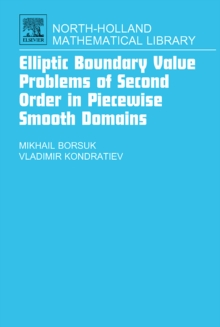Supporting your high street Find out how »
• My AccountElliptic Boundary Value Problems of Second Order in Piecewise Smooth Domains : Volume 69 Hardback

Part of the North-Holland Mathematical Library series

Description

The book contains a systematic treatment of the qualitative theory of elliptic boundary value problems for linear and quasilinear second order equations in non-smooth domains. The authors concentrate on the following fundamental results: sharp estimates for strong and weak solutions, solvability of the boundary value problems, regularity assertions for solutions near singular points. Key features:* New the Hardy - Friedrichs - Wirtinger type inequalities as well as new integral inequalities related to the Cauchy problem for a differential equation. * Precise exponents of the solution decreasing rate near boundary singular points and best possible conditions for this. * The question about the influence of the coefficients smoothness on the regularity of solutions. * New existence theorems for the Dirichlet problem for linear and quasilinear equations in domains with conical points. * The precise power modulus of continuity at singular boundary point for solutions of the Dirichlet, mixed and the Robin problems. * The behaviour of weak solutions near conical point for the Dirichlet problem for m - Laplacian. * The behaviour of weak solutions near a boundary edge for the Dirichlet and mixed problem for elliptic quasilinear equations with triple degeneration.

Information

• Format: Hardback
• Pages: 538 pages
• Publisher: Elsevier Science & Technology
• Publication Date:
• Category: Differential calculus & equations
• ISBN: 9780444521095

£180.00

£154.65

on all orders

Pick up orders

from local bookshops

£49.99

£40.35

£49.99

£49.99

£40.79

£49.99